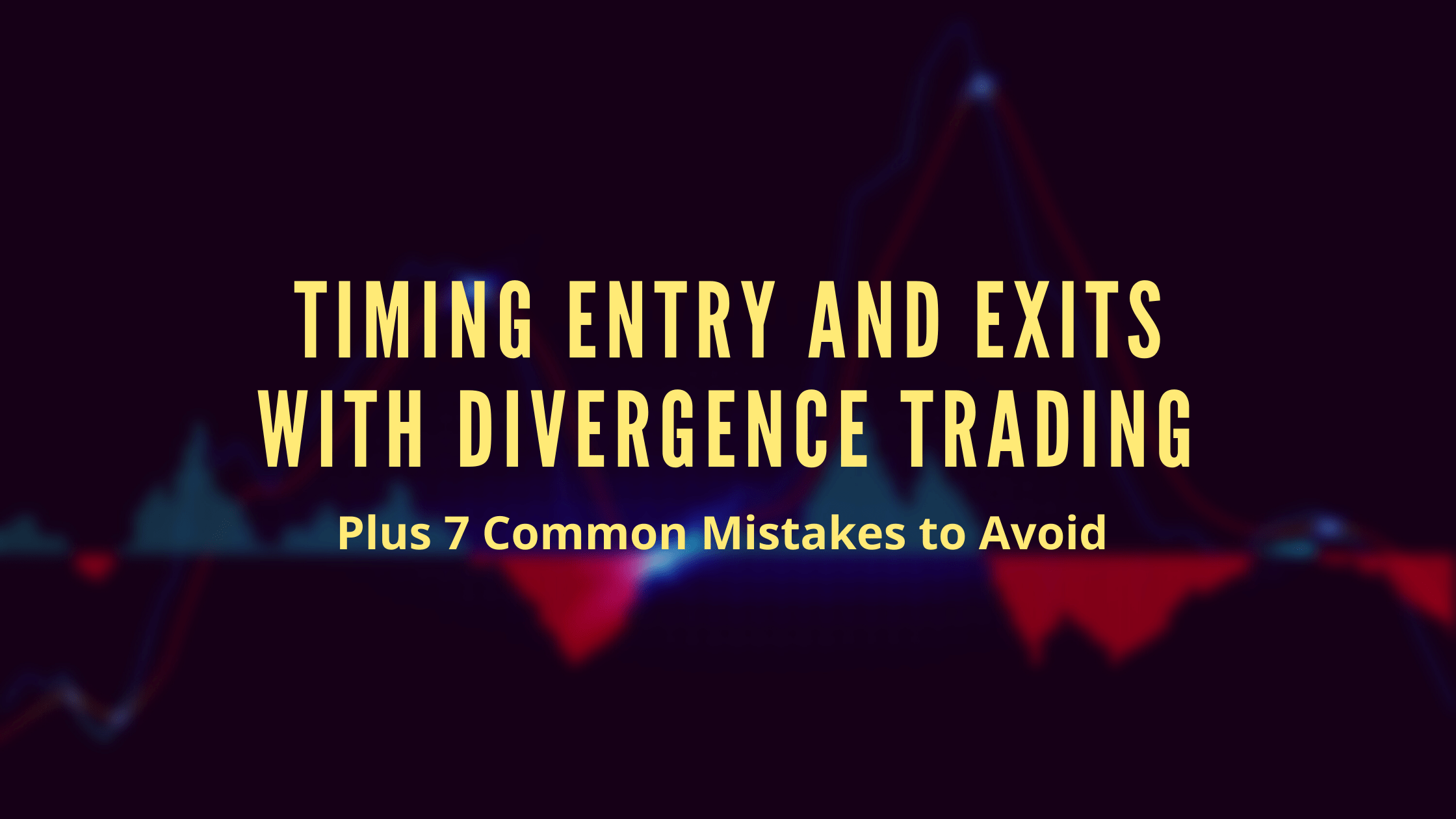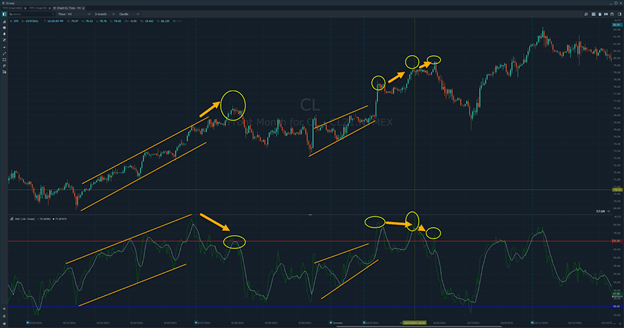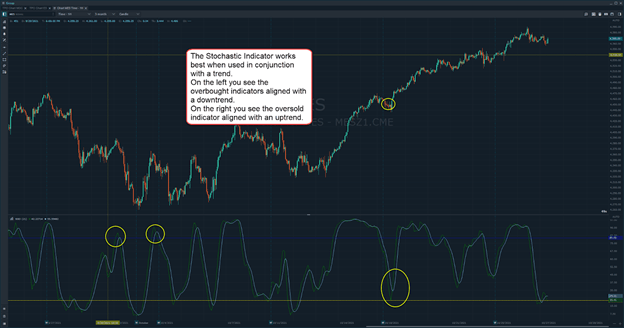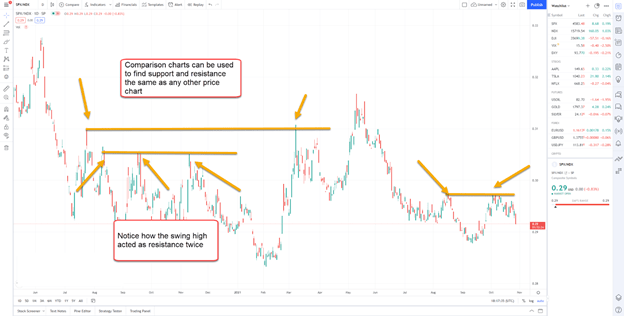Timing Entry and Exits with Divergence Trading

## Timing Entry And Exits With Divergence Trading | Plus 7 Common Mistakes to AvoidAs traders, we seek to maximize profits. Knowing when to enter or exit a trade, however, can be a challenge. Divergence trading can help a trader buy near the bottom and sell near the peak.

This article aims to explain what trading divergences are, how to spot them, the best indicators to guide you, along with a few key strategies you may not have considered.

Plus, we’ll lay out 7 common mistakes we see when trading divergences.

## What Is A Trading Divergence?

Divergence refers to a deviation from an average or norm.

For trading purposes, we’ll consider them through two areas: correlation and indicators.

So what is a correlation?

Correlations are the measure of how well two assets move together measured on a scale of -1 to +1.

• -1 means the two assets move in exactly opposite directions. A long position and short position in the micro S&P 500 futures have a -1 correlation.
• +1 means the two assets move in the exact same direction. A long position in CME Group Micro E-mini Nasdaq 100 futures and the QQQ ETF has a +1 correlation.
• 0 means the two assets move entirely independently of one another.
What we want to look for are breakdowns in this correlation or movements away from what is typical.

For example, we know that the CME Group Micro E-mini Nasdaq 100 Futures and Micro E-mini S&P 500 Futures often trade in the same direction. If one changes course, it creates a potential divergence setup.

The other type of divergence comes from indicators such as an oscillator.

An oscillator represents the momentum of a stock. If price moves in the opposite direction of the indicator’s momentum, it implies a divergence away from what is typical.

While divergence is not a strong trigger or trading signal, it is useful for understanding the underlying momentum in an asset’s price.

## How To Spot Divergence In Futures Trading

Identifying divergences can be tricky.

Many traders will dig deeper into ideas such as implicit divergences, as part of a larger strategy.

Yet, divergences are as we said earlier: a deviation from the norm.

When we look at correlated assets, such as the Micro E-mini Nasdaq 100 futures and Micro E-mini S&P 500 Futures, we want to identify points when we expect the divergences to collapse back to the average. This is known as mean reversion.

Think of it this way.

Let’s say the Micro E-mini Nasdaq 100 futures and Micro E-mini S&P 500 Futures have a correlation of 0.8. That means the two trade in the same direction most of the time.

We come across a period where the Micro E-mini Nasdaq 100 futures has gained 3% over the last two days yet the Micro E-mini S&P 500 Futures have remained flat.

Under normal conditions, we would expect the Micro E-mini S&P 500 Futures to be up say 2%.

So, we look at this gap between the two as a divergence and can place a trade expecting either the Micro E-mini S&P 500 Futures to catch up to the Micro E-mini Nasdaq 100 Futures.

Indicator divergences look for differences between price action and an indicators signal or within the indicator itself. They attempt to identify either changes or continuations in momentum.

For example, in the chart below, you can see the Crude oil futures and the Relative Strength Index (RSI) plotted on the bottom.The RSI measures relative overbought or oversold strength.

In the chart above, you can see two instances of the same setup. The RSI and Crude futures make a series of higher highs and higher lows.

While futures continue to make a higher high, the RSI makes a lower high. This divergence creates a potential trend reversal.

## Best Divergence Indicators

There are four commonly used divergence indicators.

### 1: MACD

The Moving Average Convergence Divergence (MACD) indicator has three components: A slow-moving average, a fast-moving average, and the difference between the two.

Oftentimes, the moving averages are plotted as lines while the difference between the two is plotted as a histogram.

The basic rub is that the slower moving average takes more time to react than the faster one. When we compare this to the price of an asset, we look to see if the MACD tells us anything about the short-term (fast-moving average) trend compared to the medium-term (slow moving average) trend.### 2: RSI

The Relative Strength Index (RSI) is an oscillator indicator with one line that vacillates between three different regions: oversold, overbought, and neutral.

Relative Strength (RS) is calculated by taking the average of the most recent gains and dividing that number by the average of the most recent losses. We then convert it into a range from 0-100.

The oversold area represents the space below 30, the overbought zone is the space greater than 70, and the neutral zone is the area between the 30 and 70 lines.

You can use this as a measure of current momentum on the stock to go with a trend or predict a reversal.

An example is listed in the preceding section.

### 3: Stochastic Indicator

The stochastic indicator measures the price of an asset compared to its recent trading range.

Like RSI, the stochastic indicator has two lines that fluctuate between 0 and 100. The difference is the oversold zone Is below 20 and the overbought zone above 80, with the neutral zone between 20 and 80.

However, the stochastic indicator assumes the closing prices continue the current trend. RSI looks for short-term reversals using overbought and oversold conditions by measuring the velocity of price movements.### 4: Commodity Channel Index

CCI measures the difference between the current price and the historical average price. While it functions similarly to RSI, it doesn’t have a predefined range.

When the indicator is above the zero line, it means the asset is trading above its historical average and the opposite when it’s trading below the historical average.

Typically, if the indicator line rises above 100, it gives a sell signal, whereas if the indicator line drops below -100, it shows a buy signal

The Commodity Chanel Index (CCI), which was originally developed as a commodity trading indicator, consists of an indicator line, a zero line, and overbought & oversold areas.## How To Time Entries And Exits With Divergence Trading

We first want to point out a key concept – Strategies to timing an exit are the same as strategies to time an entry.

Think about that for a moment.

Divergences are meant to highlight points where the price or trend changes. You can use those for an entry or an exit in a trade. They’re just different sides of the same coin.

The RSI trade is one we alluded to earlier.

In this trade, you look for a change in the patterns of how the RSI and an asset have been trading together.

Here are the basics of the plan:

1. Find an asset that is trending in a given direction.
2. Look for a pattern in the asset’s price and the RSI.
3. Locate a point where the two deviate from that pattern.
4. Use that to formulate the entry or exit point.

Let’s use the crude oil futures chart from earlier as an example.We can see how in both cases the price of crude oil is in an upward trend, making a series of higher highs and higher lows.

Below that, the RSI makes the exact same pattern…

That is until we get to the divergence point.

In the areas noted with yellow circles, while crude oil futures makes a higher high, the RSI makes a lower high.

That says the upward momentum may be waning and creates a potential setup for a short-term trade in the opposite direction.

### Markets Mean Reversion

As we discussed earlier, two highly correlated markets can be used to create a mean reversion trade.

With this trade, you’re looking for points where the difference in price between the two moves outside of statistical norms.

Let’s take a look at the S&P 500 vs the Nasdaq 100.

First, we want to show you the general trend of the two over time.This chart plots the daily range of the S&P 500 divided by the Nasdaq 100.

As you can see, the Nasdaq 100 outperformed the S&P 500 over time.

Now, let’s zoom in a bit.What most people don’t realize is you can take charts like these and still use common technical analysis.

Here, we know that the long-term trend is downward.

So, when the spread rises, we can look for resistance levels using swing points the same as we would with any other chart.

At these extremes, a trader can sell the S&P 500 against the Nasdaq 100 and wait until the typical spread reverts back towards the mean or a support level.

For more on Divergence Trading, check Optimus Futures’ weekly trading strategies on TradingView, which looks for mean reversion between Micro E-Mini S&P 500 and Micro E-Mini Nasdaq 100 futures.

As we noted earlier, the stochastics works best at finding short-term trend reversals within a larger trend.

For the bullish divergence trade, we want to find a spot where the price is:

1. In a longer-term uptrend
2. Created a short-term pullback
3. Ready to reverse back to continue the longer-term trend

The chart of the E-micro Dow Futures below illustrates a perfect example.At the left side of the chart, you see price make a lower low along with the stochastic indicator.

Later on, we see price making a lower low but the stochastic making a higher low.

This divergence is within a larger trend higher.

## 7 Mistakes to Avoid When You Trade Divergence

1. Only look for divergences during trending markets – momentum indicators don’t work well during range-bound markets
2. Use momentum-based indicators such as oscillators. Lagging indicators such as moving averages don’t work for divergences.
3. Always look for confluence by consulting other tools or concepts.
4. Remember, divergences are meant to signal reversals.
5. Stick with one type of momentum indicator at a time.
6. Don’t assume that because you will get an entry signal for a trade based on divergences that you will get one for the exit as well.
7. Make sure the indicator and the chart are set to the same timeframe.